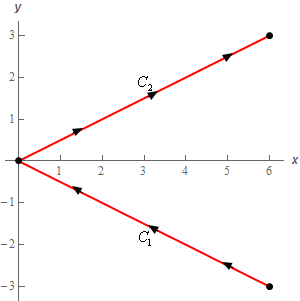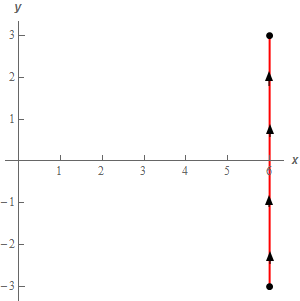Paul's Online Notes
Home / Calculus III / Line Integrals / Line Integrals of Vector Fields
Show Mobile Notice Show All Notes Hide All Notes
Mobile Notice
You appear to be on a device with a "narrow" screen width (i.e. you are probably on a mobile phone). Due to the nature of the mathematics on this site it is best views in landscape mode. If your device is not in landscape mode many of the equations will run off the side of your device (should be able to scroll to see them) and some of the menu items will be cut off due to the narrow screen width.

### Section 16.4 : Line Integrals of Vector Fields

7. Evaluate $$\displaystyle \int\limits_{C}{{\vec F\centerdot d\vec r}}$$ where $$\vec F\left( {x,y} \right) = \left( {6x - 2y} \right)\,\vec i + {x^2}\vec j$$ for each of the following curves.

1. $$C$$ is the line segment from $$\left( {6, - 3} \right)$$ to $$\left( {0,0} \right)$$ followed by the line segment from $$\left( {0,0} \right)$$ to $$\left( {6,3} \right)$$.
2. $$C$$ is the line segment from $$\left( {6, - 3} \right)$$ to $$\left( {6,3} \right)$$.
Show All Solutions Hide All Solutions

a $$C$$ is the line segment from $$\left( {6, - 3} \right)$$ to $$\left( {0,0} \right)$$ followed by the line segment from $$\left( {0,0} \right)$$ to $$\left( {6,3} \right)$$. Show All Steps Hide All Steps
Start Solution

Let’s start off with a quick sketch of the curve for this part of the problem.Here is the parameterization for each of these curves.

$${C_1}:\,\,\vec r\left( t \right) = \left( {1 - t} \right)\left\langle {6, - 3} \right\rangle + t\left\langle {0,0} \right\rangle = \left\langle {6 - 6t, - 3 + 3t} \right\rangle \hspace{0.25in}\,\,\,\,\,\,\,0 \le t \le 1$$
$${C_2}:\,\,\vec r\left( t \right) = \left\langle {t,\frac{1}{2}t} \right\rangle \hspace{0.25in}\,\,\,\,\,\,\,0 \le t \le 6$$

For $${C_2}$$ we used the equation of the line to get the parameterization because it gave a slightly nicer form to work with. We couldn’t do this with $${C_1}$$ because the specified direction of the curve was in the decreasing $$x$$ direction and the limits of the integral need to be from smaller value to larger value.

Show Step 2

Here is the line integral for each of these curves.

\begin{align*}\int\limits_{{{C_1}}}{{\vec F\centerdot d\vec r}} & = \int_{0}^{1}{{\left\langle {6\left( {6 - 6t} \right) - 2\left( { - 3 + 3t} \right),{{\left( {6 - 6t} \right)}^2}} \right\rangle \centerdot \left\langle { - 6,3} \right\rangle \,dt}}\\ & = \int_{0}^{1}{{252t - 252 + 3{{\left( {6 - 6t} \right)}^2}\,dt}}\\ & = \left. {\left( {126{t^2} - 252t - \frac{1}{6}{{\left( {6 - 6t} \right)}^3}} \right)} \right|_0^1 = \underline { - 90} \end{align*} \begin{align*}\int\limits_{{{C_2}}}{{\vec F\centerdot d\vec r}} & = \int_{0}^{6}{{\left\langle {6\left( t \right) - 2\left( {\frac{1}{2}t} \right),{{\left( t \right)}^2}} \right\rangle \centerdot \left\langle {1,\frac{1}{2}} \right\rangle \,dt}}\\ & = \int_{0}^{6}{{5t + \frac{1}{2}{t^2}\,dt}} = \left. {\left( {\frac{5}{2}{t^2} + \frac{1}{6}{t^3}} \right)} \right|_0^6 = \underline {126} \end{align*} Show Step 3

The line integral for this part is then,

$\int\limits_{C}{{\vec F\centerdot d\vec r}} = \left( { - 90} \right) + \left( {126} \right) = \require{bbox} \bbox[2pt,border:1px solid black]{{36}}$

b $$C$$ is the line segment from $$\left( {6, - 3} \right)$$ to $$\left( {6,3} \right)$$. Show All Steps Hide All Steps
Start Solution

Let’s start off with a quick sketch of the curve for this part of the problem.So, what we have in this part is a different curve that goes from $$\left( {6, - 3} \right)$$ to $$\left( {6,3} \right)$$. Despite the fact that this curve has the same starting and ending point as the curve in the first part there is no reason to expect the line integral to have the same value. Therefore, we’ll need to go through the work and see what we get from the line integral.

We’ll need to parameterize the curve so let’s take care of that.

$$C:\,\,\vec r\left( t \right) = \left\langle {6,t} \right\rangle \hspace{0.25in}\,\,\,\,\,\,\, - 3 \le t \le 3$$

Show Step 2

Now all we need to do is compute the line integral.

\begin{align*}\int\limits_{C}{{\vec F\centerdot d\vec r}} & = \int_{{ - 3}}^{3}{{\left\langle {6\left( 6 \right) - 2\left( t \right),{{\left( 6 \right)}^2}} \right\rangle \centerdot \left\langle {0,1} \right\rangle \,dt}}\\ & = \int_{{ - 3}}^{3}{{36\,dt}} = \left. {\left( {36t} \right)} \right|_{ - 3}^3 = \require{bbox} \bbox[2pt,border:1px solid black]{{216}}\end{align*}

So, as noted at the start of this part the value of the line integral was not the same as the value of the line integral in the first part despite the same starting and ending points for the curve. Note that it is possible for two line integrals with the same starting and ending points to have the same value but we can’t expect that to happen and so need to go through and do the work.